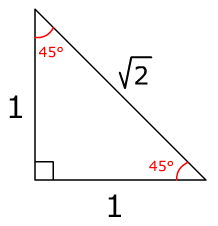# Quick method to know that the shorter side angles at the end were 4 sqrt 2?

• Module 2 Week 1 Day 2 Your Turn Explanation Part 2

Was there some sort of quick method that Prof. Loh used to know that the shorter side angles at the end were 4 sqrt 2?

• Hi TSS Graviser,

Here we are looking at a right triangle with two 45° angles. For this type of triangle (45°-45°-90° triangle), the ratio of the side lengths is always 1:1:$$\sqrt{2}$$, $$\sqrt{2}$$ being the length of the hypotenuse. So in this case, since the hypotenuse is 8 you can set up a ratio equation like this (x = length of a leg of the triangle):
$$\frac{\sqrt{2}}{1} = \frac{8}{x}$$
Cross-multiplying,
$$\sqrt{2} \times x = 8\times1$$
Dividing both sides by $$\textcolor{red}{\sqrt{2}}$$ (Remember this step because it's very important),
$$x = \frac{8\times1}{\sqrt{2}} = \frac{8}{\sqrt{2}}$$
Multiplying both sides by $$\frac{\sqrt{2}}{\sqrt{2}}$$ (which is equal to 1),
$$\frac{\sqrt{2}}{\sqrt{2}} \times x = \frac{8}{\sqrt{2}} \times \frac{\sqrt{2}}{\sqrt{2}}$$
$$x = \frac{8\sqrt{2}}{2} = 4\sqrt{2}$$
Therefore, the length of each leg of the triangle is $$4\sqrt{2}$$.

Now, let's think: What did we do to get $$4\sqrt{2}$$?

We divided 8 by $$\sqrt{2}$$! This is why I said that step above is very important. This is also why Prof. Loh says "this side is then 8 divided by the square root of 2" when he writes $$4\sqrt{2}$$ as the legs' length in the video. This idea applies to all 45-45-90 triangles. For example, if the hypotenuse of a 45-45-90 triangle is 16, we would divide 16 by $$\sqrt{2}$$ to calculate the side length of the legs.

Hope this helped!

If you are not familiar with the concept of ratios, here's another explanation. You can also use a simple triangle with 2 legs of length 1 and a hypotenuse of $$\sqrt{2}$$, which is shown below:Using the idea of similar triangles, you can determine the leg lengths of the triangle with the hypotenuse of 8 by figuring out by how much the triangle is scaled up from the simple triangle. In other words, what number do you have to multiply to $$\sqrt{2}$$ in order to get 8? Let's call this mystery number $$k$$ and solve for it!
$$\sqrt{2} \times k = 8$$
Dividing both sides by $$\textcolor{red}{\sqrt{2}}$$ (Remember this step because it's very important),
$$k = \frac{8}{\sqrt{2}}$$
Multiplying both sides by $$\frac{\sqrt{2}}{\sqrt{2}}$$ (which is equal to 1),
$$\frac{\sqrt{2}}{\sqrt{2}} \times k = \frac{8}{\sqrt{2}} \times \frac{\sqrt{2}}{\sqrt{2}}$$
$$k = \frac{8\sqrt{2}}{2} = 4\sqrt{2}$$
Therefore, the side lengths of the triangle with a hypotenuse of 8 is scaled up from the simple triangle by a factor of $$4\sqrt{2}$$. This means for the rest of the sides of the triangle, you just need to multiply the side lengths of the simple triangle by $$4\sqrt{2}$$!

Since we know the length of the hypotenuse already, we just need to find the length of the two legs, which have the same lengths, as shown in the figure above. The legs of the simple triangle have a length of 1, so if you multiply that by $$4\sqrt{2}$$ you still get $$4\sqrt{2}$$.

Now, let's think: What did we do to get $$4\sqrt{2}$$?

We divided 8 by $$\sqrt{2}$$! This is why I said that step above is very important. This is also why Prof. Loh says "this side is then 8 divided by the square root of 2" when he writes $$4\sqrt{2}$$ as the legs' length in the video. This idea applies to all 45°-45°-90° triangles. For example, if the hypotenuse of a 45°-45°-90° triangle is 16, we would divide 16 by $$\sqrt{2}$$ to calculate the side length of the legs.

Hope this helped!

Prof. Loh actually explains this in the Day 2: Challenge Explanation video, so you can watch it to get a better understanding!

Happy Learning,

The Daily Challenge Team

• @minji This explanation is really clear. It makes me understand the geometry concepts in an algebraical way. Thanks!